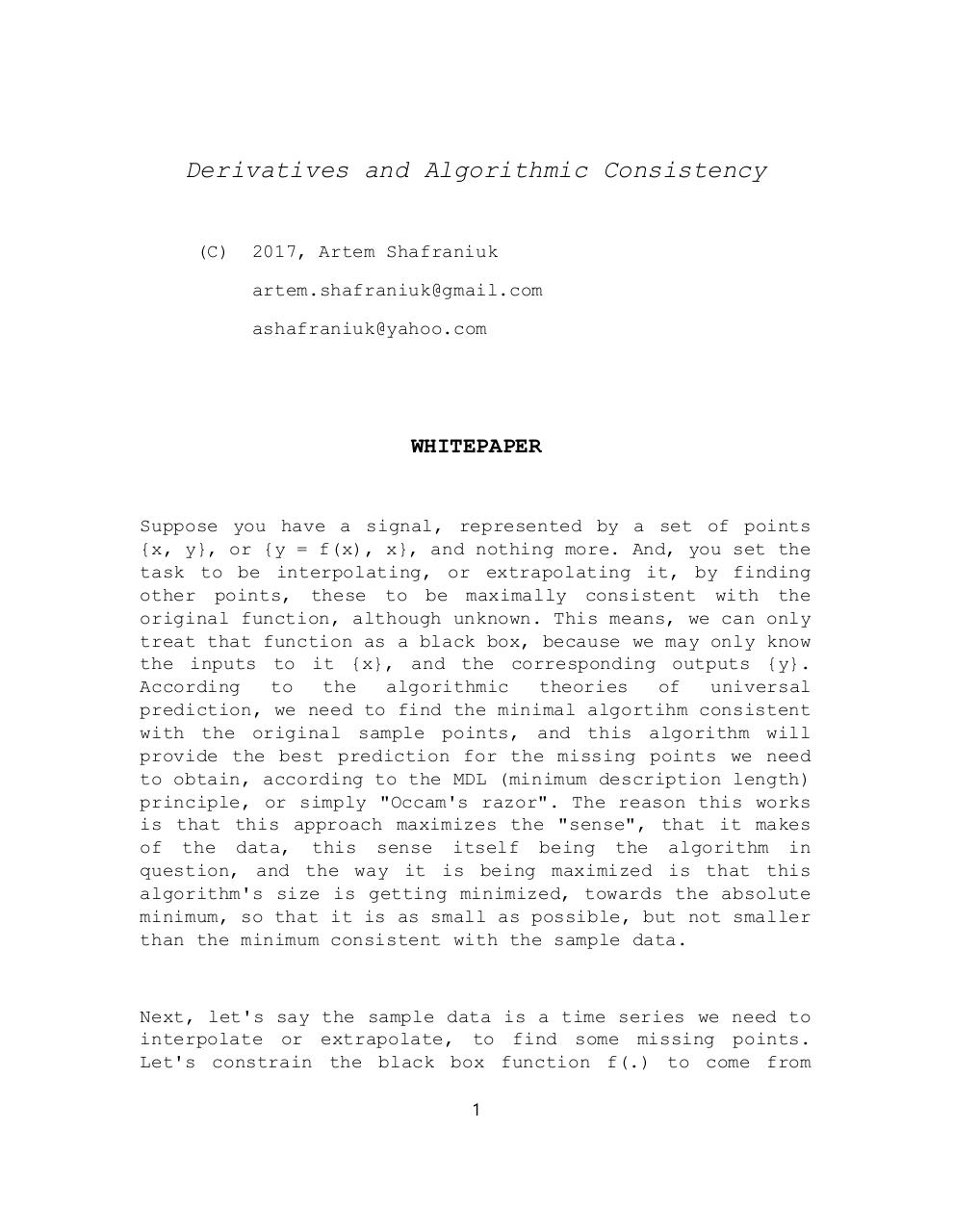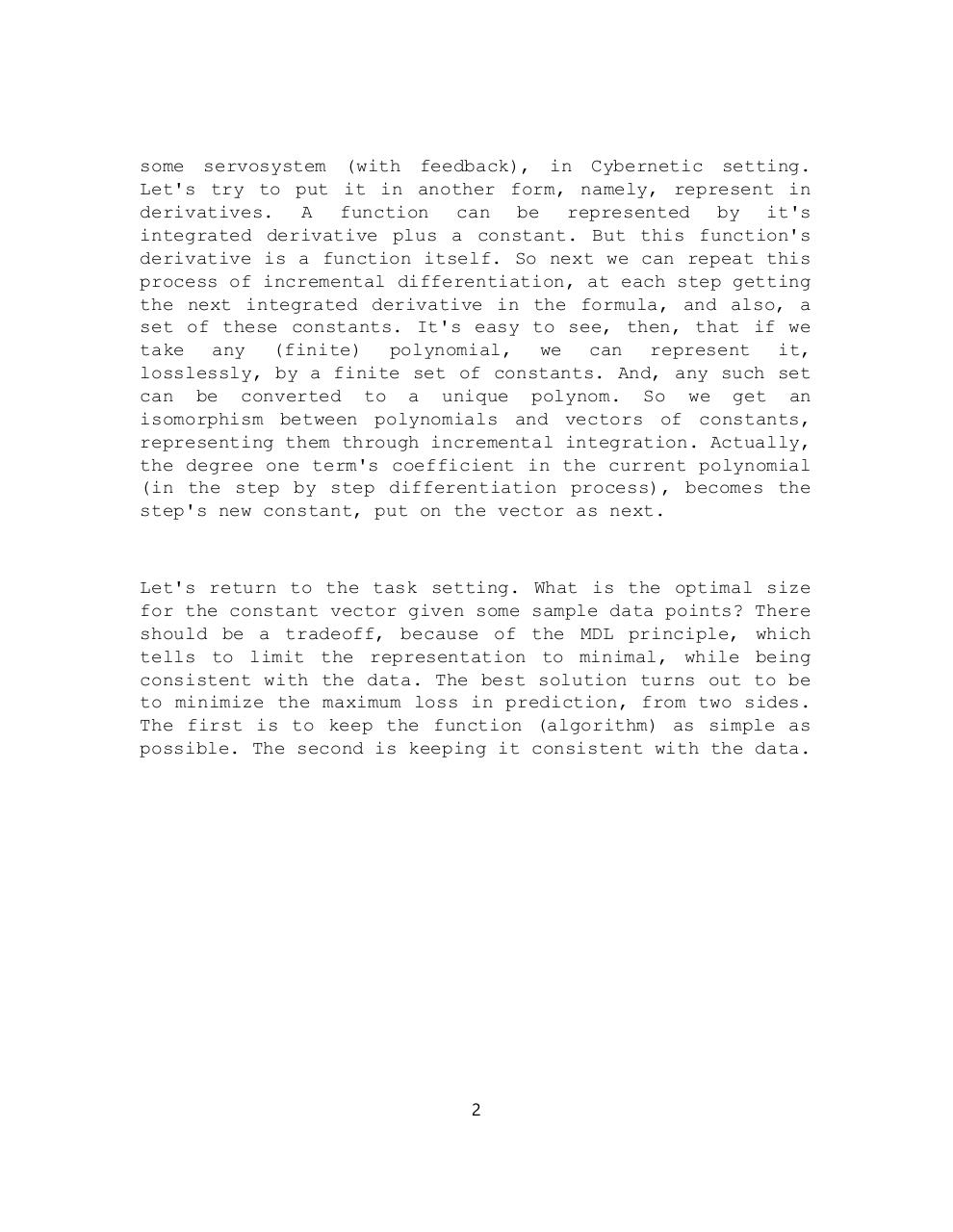# algcons .pdf

### File information

Original filename: algcons.pdf
Title: ArtemDACP
Author: artem

This PDF 1.7 document has been generated by / Microsoft: Print To PDF, and has been sent on pdf-archive.com on 19/04/2017 at 18:34, from IP address 107.135.x.x. The current document download page has been viewed 279 times.
File size: 218 KB (2 pages).
Privacy: public file

algcons.pdf (PDF, 218 KB)

### Document preview

Derivatives and Algorithmic Consistency
(C)

2017, Artem Shafraniuk
artem.shafraniuk@gmail.com
ashafraniuk@yahoo.com

WHITEPAPER

Suppose you have a signal, represented by a set of points
{x, y}, or {y = f(x), x}, and nothing more. And, you set the
task to be interpolating, or extrapolating it, by finding
other points, these to be maximally consistent with the
original function, although unknown. This means, we can only
treat that function as a black box, because we may only know
the inputs to it {x}, and the corresponding outputs {y}.
According
to
the
algorithmic
theories
of
universal
prediction, we need to find the minimal algortihm consistent
with the original sample points, and this algorithm will
provide the best prediction for the missing points we need
to obtain, according to the MDL (minimum description length)
principle, or simply "Occam's razor". The reason this works
is that this approach maximizes the "sense", that it makes
of the data, this sense itself being the algorithm in
question, and the way it is being maximized is that this
algorithm's size is getting minimized, towards the absolute
minimum, so that it is as small as possible, but not smaller
than the minimum consistent with the sample data.

Next, let's say the sample data is a time series we need to
interpolate or extrapolate, to find some missing points.
Let's constrain the black box function f(.) to come from
1

some servosystem (with feedback), in Cybernetic setting.
Let's try to put it in another form, namely, represent in
derivatives. A function can be represented by it's
integrated derivative plus a constant. But this function's
derivative is a function itself. So next we can repeat this
process of incremental differentiation, at each step getting
the next integrated derivative in the formula, and also, a
set of these constants. It's easy to see, then, that if we
take any (finite) polynomial, we can represent it,
losslessly, by a finite set of constants. And, any such set
can be converted to a unique polynom. So we get an
isomorphism between polynomials and vectors of constants,
representing them through incremental integration. Actually,
the degree one term's coefficient in the current polynomial
(in the step by step differentiation process), becomes the
step's new constant, put on the vector as next.

for the constant vector given some sample data points? There
should be a tradeoff, because of the MDL principle, which
tells to limit the representation to minimal, while being
consistent with the data. The best solution turns out to be
to minimize the maximum loss in prediction, from two sides.
The first is to keep the function (algorithm) as simple as
possible. The second is keeping it consistent with the data.

2#### HTML Code

Copy the following HTML code to share your document on a Website or Blog

#### QR Code### Related keywords